# Problem 52562. Easy Sequences 6: Coefficient sums of derivatives

Consider the polynomial function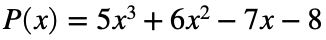and its first-order derivative. The sums of the coefficients of P and P', are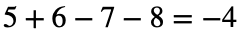and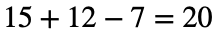, respectively. If we keep summing up coefficients for all higher derivatives the sums sequence will be as follows: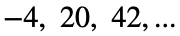etc. The total sum of this sequence converge to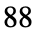.
For this exercise, you are given an array corresponding to the coefficients of a polynomial function. In the example above, the coefficient array is therefore,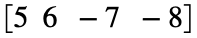. Your task is to find the total of the sum of the coefficients of the given polynomial function plus the sum of the coefficients of its first derivative plus the sum of cefficients of all its higher degree derivatives.

### Solution Stats

11.86% Correct | 88.14% Incorrect
Last Solution submitted on Oct 29, 2023

### Community Treasure Hunt

Find the treasures in MATLAB Central and discover how the community can help you!

Start Hunting!